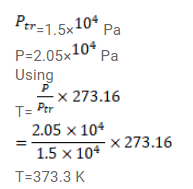# A constant volume thermometer registers a pressure ofQuestion:

A constant volume thermometer registers a pressure of $1.500 \times 10^{4} \mathrm{~Pa}$ at the triple point of water and a pressure of $2.050 \times 10^{4} \mathrm{~Pa}$ at the normal boiling point. What is the temperature at the normal boiling point?

Solution: